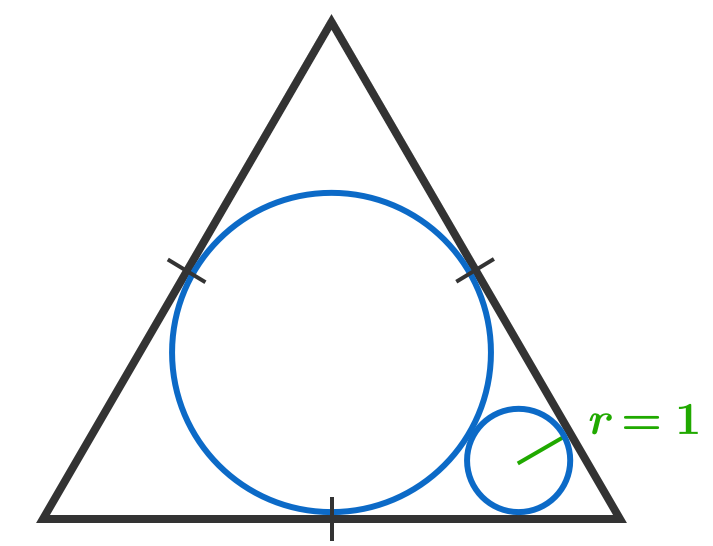# Observable Planets

Geometry Level 2A circle is inscribed in an equilateral triangle. A smaller circle is drawn such that it is tangent to two sides of the triangle as well as the larger circle.

The area of the equilateral triangle is $a \sqrt{b}$, where $a$ and $b$ are positive integers with $b$ square-free. Find $a + b.$

×